# Remez tutorial 3/5: changing variables for simpler polynomials

In the previous section, we introduced function g(x) and saw that RemezSolver looked for a polynomial P(x) and the smallest error value E such that: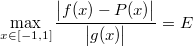Where g(x) is a function used as a weight to minimise relative error.

We will now see that g(x) can be used for other purposes.

## Odd and even functions

An odd function is such that in all points, f(-x) = -f(x). Similarly, an even function is such that f(-x) = f(x).

An example of an odd function is sin(x). An example of an even function is cos(x).

A reasonable expectation is that approximating an odd function over an interval centred at zero will lead to an odd polynomial. But due to the way the Remez exchange algorithm works, this is not necessarily the case. We can help the algorithm a little.

## The example of sin(x)

Suppose we want to approximate sin(x) over [-π/2; π/2]: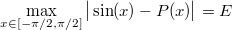We know sin(x) is an odd function, so instead we look for an odd polynomial. Odd polynomials are polynomials that only use odd powers of x, such as x, , x⁵

We notice that an odd polynomial P(x) can always be rewritten as xQ(x²) where Q has the same coefficients. Our minimax constraint becomes: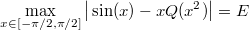Another observation is that replacing x with -x has no effect on the expression in the left. We can therefore restrict the range to [0; π/2]:Now since x is always positive, we can at last perform our change of variable, replacing with y (and x with √y̅, since x is positive), and changing the range accordingly: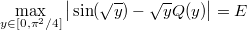This is almost something RemezSolver can deal with, if only there wasn’t this √y̅ in front of Q(y). Fortunately we can simply divide everything by √y̅: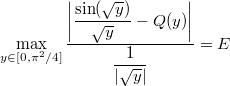That’s it! We have our minimax equation, and we can feed it to RemezSolver.

## Source code

#include "lol/math/real.h"
#include "lol/math/remez.h"

using lol::real;
using lol::RemezSolver;

real f(real const &y)
{
real sqrty = sqrt(y);
return sin(sqrty) / sqrty;
}

real g(real const &y)
{
return re(sqrt(y));
}

int main(int argc, char **argv)
{
RemezSolver<4, real> solver;
solver.Run("1e-1000", real::R_PI_2 * real::R_PI_2, f, g, 40);
return 0;
}


Several subtelties here:

• sqrt(y) is computed only once, to save computations
• f is not defined at zero; instead of defining it, we simply reduce the range to [1e-1000; π²/4] instead of [0; π²/4]
• since 1e-1000 is too small for any C++ literal number, we must use a string representation

## Compilation and execution

Build and run the above code:

make
./remez


After all the iterations the output should be as follows:

Step 8 error: 3.338112377353099148424378937190071485401e-9
Polynomial estimate:
x**0*9.999999765898820673279342160490060830302e-1
+x**1*-1.666664763463971252758602707042821974958e-1
+x**2*8.332899823351751253473706862398940753675e-3
+x**3*-1.980089776279543126829999863143134719418e-4
+x**4*2.590488500536052274124208263889095025208e-6


We can therefore write the corresponding C++ function:

double fastsin(double x)
{
const double a1 = 9.999999765898820673279342160490060830302e-1;
const double a3 = -1.666664763463971252758602707042821974958e-1;
const double a5 = 8.332899823351751253473706862398940753675e-3;
const double a7 = -1.980089776279543126829999863143134719418e-4;
const double a9 = 2.590488500536052274124208263889095025208e-6;

return x * (a1 + x*x * (a3 + x*x * (a5 + x*x * (a7 + x*x * a9))));
}


Note that because of our change of variables, the polynomial coefficients are a1, a3, a5… instead of a0, a1, a2

## Analysing the results

The obtained polynomial needs 5 constants for a maximum error of about 3.3381e-9 over [-π/2; π/2]. The real 9th degree minimax polynomial on [-π/2; π/2] needs 10 constants for a maximum error of about… 3.3381e-9!Again, the error curves match almost perfectly. It means that the odd coefficients in the minimax approximation of an even function play very little role.

## Conclusion

You should now be able to give hints to the Remez solver through changes of variables, and find polynomials with fewer constants!

Please report any trouble you may have had with this document to sam@hocevar.net. You may then carry on to the next section: fixing lower-order parameters.